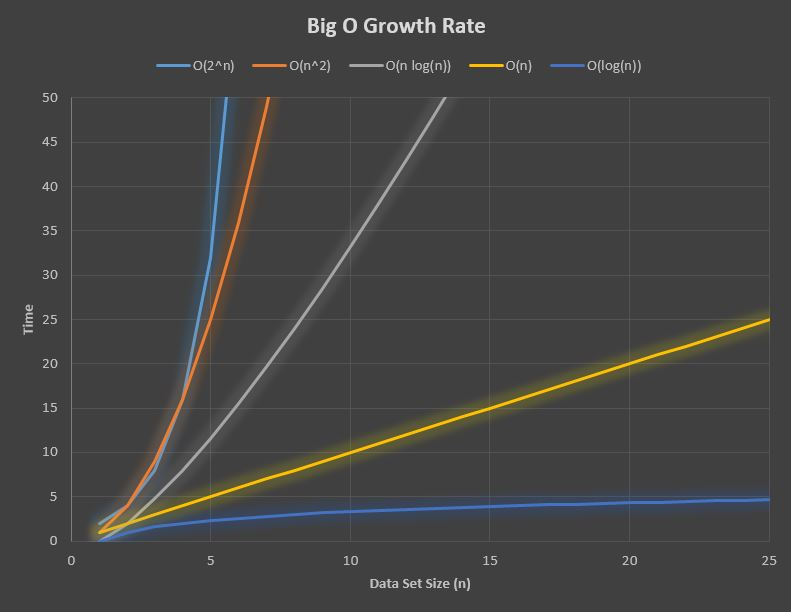Java Chapter 16 - Big O Notation

In comparing algorithms (e.g. sorts), the Big O Notation can be used to compare their growth rate as the size of the data set (n) increases.  Big O is just one tool to compare algorithms.  Just because two algorithms have the same Big O doesn't mean that they are exactly equivalent - there are other variables to consider.

Big O typically shows how many iterations (loops) are necessary and is expressed in terms of the data set size n.  It states the worst case scenario.  For example, a for loop would be listed as
O(n) whereas a nested for loop would be O(n2).

16
.1  Big O Examples

The Big O notation can be used to compare the complexity or growth rate of algorithms.  It gives you the growth rate for the worst case scenario.  Here are some examples:

 Big O Description Example(s) O(1) This describes an algorithm that will execute in the same amount of time regardless of the size of the data input. - A series of if statements or a fixed size loop O(n) This describes an algorithm the grows linearly in proportion to the size of the data set (n). - a for loop that executes n times - Searching an array or stack O(n2) This describes an algorithm that grows proportionally to the square of the size of the data set.  A nested for loop such as the bubble sort has this growth rate. - nested for loop - bubble sort - selection sort O(log(n)) This describes an algorithm that grows logarithmically to the size of the data set.  Note that in programming this often means a base logarithm: log2(n) - binary search tree O(n log(n)) This describes an algorithm where the data set is split in halves recursively and each part is executed n times. - merge sort

For example, the Bubble Sort can be written as a nested for loop where each loop executes n times (below).  Therefore, the growth rate is the square of the growth rate of the size of the data set (n).  This is written
O(n2).

// bubble sort
for (int x=0; x<n; x++)
{
for (int y=0; y<n; y++)
{

// compare data[y] & data[y+1] and swap if needed
}
}

The graph below shows the growth rate for some popular algorithms.  The y-axis is usually expressed as time, but could also be expressed as number of iterations.16
.2  Algorithms Compared

 Algorithm Big O Space Complexity Array Search O(n) O(n) Stack Search O(n) O(n) Linked List Search O(n) O(n) Binary Tree Search O(log(n)) O(n) Bubble Sort O(n2) O(n) Selection Sort O(n2) O(n) Merge Sort O(n log(n)) O(log(n)) Radix Sort O(nk) O(n+k)

n = dataset size,  k = length of keys